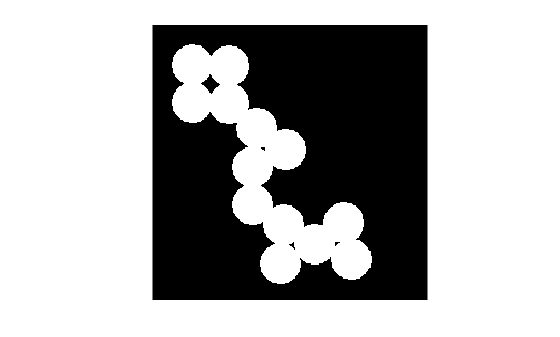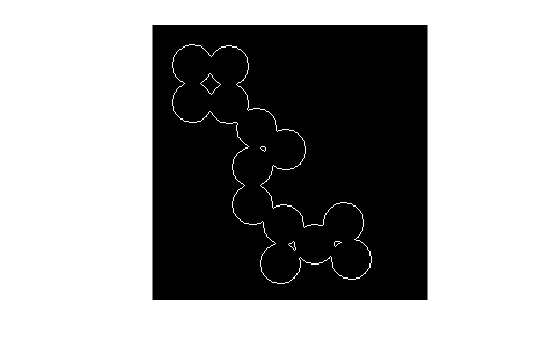# bwmorph

## 语法

``BW2 = bwmorph(BW,operation)``
``BW2 = bwmorph(BW,operation,n)``

## 说明

````BW2 = bwmorph(BW,operation)` 对二值图像 `BW` 应用特定的形态学运算。 注意要对三维体图像执行形态学运算，请使用 `bwmorph3`。 ```
``BW2 = bwmorph(BW,operation,n)` 应用 `n` 次运算。`n` 可以是 `Inf`，在这种情况下会一直重复运算，直到图像不再变化。`

## 示例

```BW = imread('circles.png'); imshow(BW);``````BW2 = bwmorph(BW,'remove'); figure imshow(BW2)``````BW3 = bwmorph(BW,'skel',Inf); figure imshow(BW3)```## 输入参数

`'bothat'`

`bwmorph` 函数使用邻域 `ones(3)` 执行形态学闭运算。如果要使用不同邻域执行形态学底帽运算，则请使用 `imbothat` 函数。

`'branchpoints'`

```0 0 1 0 0 0 0 0 0 0 0 0 1 0 0 0 0 0 0 0 1 1 1 1 1 becomes 0 0 1 0 0 0 0 1 0 0 0 0 0 0 0 0 0 1 0 0 0 0 0 0 0```

`'bridge'`

```1 0 0 1 1 0 1 0 1 becomes 1 1 1 0 0 1 0 1 1 ```

`'clean'`

```0 0 0 0 1 0 0 0 0 ```

`'close'`

`bwmorph` 函数使用邻域 `ones(3)` 执行形态学闭运算。如果要使用不同邻域执行形态学闭运算，则请使用 `imclose` 函数。

`'diag'`

```0 1 0 0 1 0 1 0 0 becomes 1 1 0 0 0 0 0 0 0 ```

`'endpoints'`

```1 0 0 0 1 0 0 0 0 1 0 0 becomes 0 0 0 0 0 0 1 0 0 0 1 0 0 0 0 0 0 0 0 0```

`'fill'`

```1 1 1 1 0 1 1 1 1 ```

`'hbreak'`

```1 1 1 1 1 1 0 1 0 becomes 0 0 0 1 1 1 1 1 1 ```

`'majority'`

`'open'`

`bwmorph` 函数使用邻域 `ones(3)` 执行形态学开运算。如果要使用不同邻域执行形态学开运算，则请使用 `imopen` 函数。

`'remove'`

`'shrink'`

`'skel'`

`'spur'`

```0 0 0 0 0 0 0 0 0 0 0 0 0 0 0 0 0 0 1 0 becomes 0 0 0 0 0 1 0 0 0 1 0 0 1 1 0 0 1 1 0 0 ```

`'thicken'`

`n = Inf` 时，通过向对象外部添加像素来加厚对象，直到先前未连通的对象实现 8 连通为止。此选项会保留欧拉数。

`'thin'`

`n = Inf` 时，通过从对象边界删除像素，将对象收缩为线。使没有孔洞的对象收缩为具有最小连通性的线，有孔洞的对象收缩为每个孔洞和外边界之间的连通环。此选项会保留欧拉数。有关详细信息，请参阅算法

`'tophat'`

`bwmorph` 函数使用邻域 `ones(3)` 执行形态学开运算。如果您要对不同邻域执行形态学顶帽运算，则请使用 `imtophat` 函数。

## 算法

1. 在第一个子迭代中，当且仅当条件 G1G2G3 全部满足时，才删除像素 p

2. 在第二个子迭代中，当且仅当条件 G1G2${G}_{3}\prime$ 全部满足时，才删除像素 p

### 条件 G1：

`${X}_{H}\left(p\right)=1$`

`${X}_{H}\left(p\right)=\sum _{i=1}^{4}{b}_{i}$`

x1x2、...、x8p 的八个邻点的值，从东侧邻点开始，按逆时针顺序编号。

### 条件 G2：

`$2\le \mathrm{min}\left\{{n}_{1}\left(p\right),{n}_{2}\left(p\right)\right\}\le 3$`

`${n}_{1}\left(p\right)=\sum _{k=1}^{4}{x}_{2k-1}\vee {x}_{2k}$`
`${n}_{2}\left(p\right)=\sum _{k=1}^{4}{x}_{2k}\vee {x}_{2k+1}$`

### 条件 G3：

`$\left({x}_{2}\vee {x}_{3}\vee {\overline{x}}_{8}\right)\wedge {x}_{1}=0$`

### 条件 G3'：

`$\left({x}_{6}\vee {x}_{7}\vee {\overline{x}}_{4}\right)\wedge {x}_{5}=0$`

 Haralick, Robert M., and Linda G. Shapiro, Computer and Robot Vision, Vol. 1, Addison-Wesley, 1992.

 Kong, T. Yung and Azriel Rosenfeld, Topological Algorithms for Digital Image Processing, Elsevier Science, Inc., 1996.

 Lam, L., Seong-Whan Lee, and Ching Y. Suen, "Thinning Methodologies-A Comprehensive Survey," IEEE Transactions on Pattern Analysis and Machine Intelligence, Vol 14, No. 9, September 1992, page 879, bottom of first column through top of second column.

 Pratt, William K., Digital Image Processing, John Wiley & Sons, Inc., 1991.